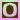Go to content
The experiment consists of tossing a coin on a floor with square tiles, and is shown graphically in the first picture box. The center (X, Y) of the coin relative to the center of the square is recorded on each update in the first table, and is shown graphically as a red dot in the scatterplot. Variable I indicates the event that the coin crosses a crack, and is recorded on each update in the first table. The density of I is shown in blue in the distribution graph, and is recorded in the distribution table. On each update, the empirical density of I is shown in red in the distribution graph, and is recorded in the distribution table. The parameter of the process is the radius r of the coin, which can be varied with the scroll bar.an applet from PSOL from UAH

## Glossary

### density

the ratio of the mass of an object to its volume

### event

any set of possible outcomes of a statistical experiment

### graph

A diagram showing a relationship between two variables.
The diagram shows a vertical y axis and a horizontal x axis.

### parameter

a) a constant or variable term in a function that determines the specific form of the function but not its general nature, as a in f(x) = ax, where a determines only the slope of the line described by f(x).
b) one of the independent variables in a set of parametric equations.

### variable

A value which is unknown and free to vary, often denoted by x or y.

Full Glossary List

## This question appears in the following syllabi:

SyllabusModuleSectionTopicExam Year
AQA A-Level (UK - Pre-2017)S1ProbabilityExperimental-
AQA AS Maths 2017StatisticsProbabilityExperimental Probabilities-
AQA AS/A2 Maths 2017StatisticsProbabilityExperimental Probabilities-
CBSE XII (India)ProbabilityProbabilityRepeated independent (Bernoulli) trials and binomial distribution-
CBSE XI (India)Statistics and ProbabilityProbabilityRandom experiments, outcomes, sample spaces (set representation)-
CCEA A-Level (NI)S1ProbabilityExperimental-
CIE A-Level (UK)S1ProbabilityExperimental-
Edexcel A-Level (UK - Pre-2017)S1ProbabilityExperimental-
Edexcel AS Maths 2017StatisticsProbabilityExperimental Probabilities-
Edexcel AS/A2 Maths 2017StatisticsProbabilityExperimental Probabilities-
I.B. Higher Level5ProbabilityExperimental-
I.B. Standard Level5ProbabilityExperimental-
Methods (UK)M15ProbabilityExperimental-
I.B. (MSSL)3ProbabilityExperimental-
OCR A-Level (UK - Pre-2017)S1ProbabilityExperimental-
OCR AS Maths 2017StatisticsProbabilityExperimental Probabilities-
OCR MEI AS Maths 2017StatisticsProbabilityExperimental Probabilities-
OCR-MEI A-Level (UK - Pre-2017)S1ProbabilityExperimental-
Pre-Calculus (US)E4ProbabilityExperimental-
Pre-U A-Level (UK)ProbProbabilityExperimental-